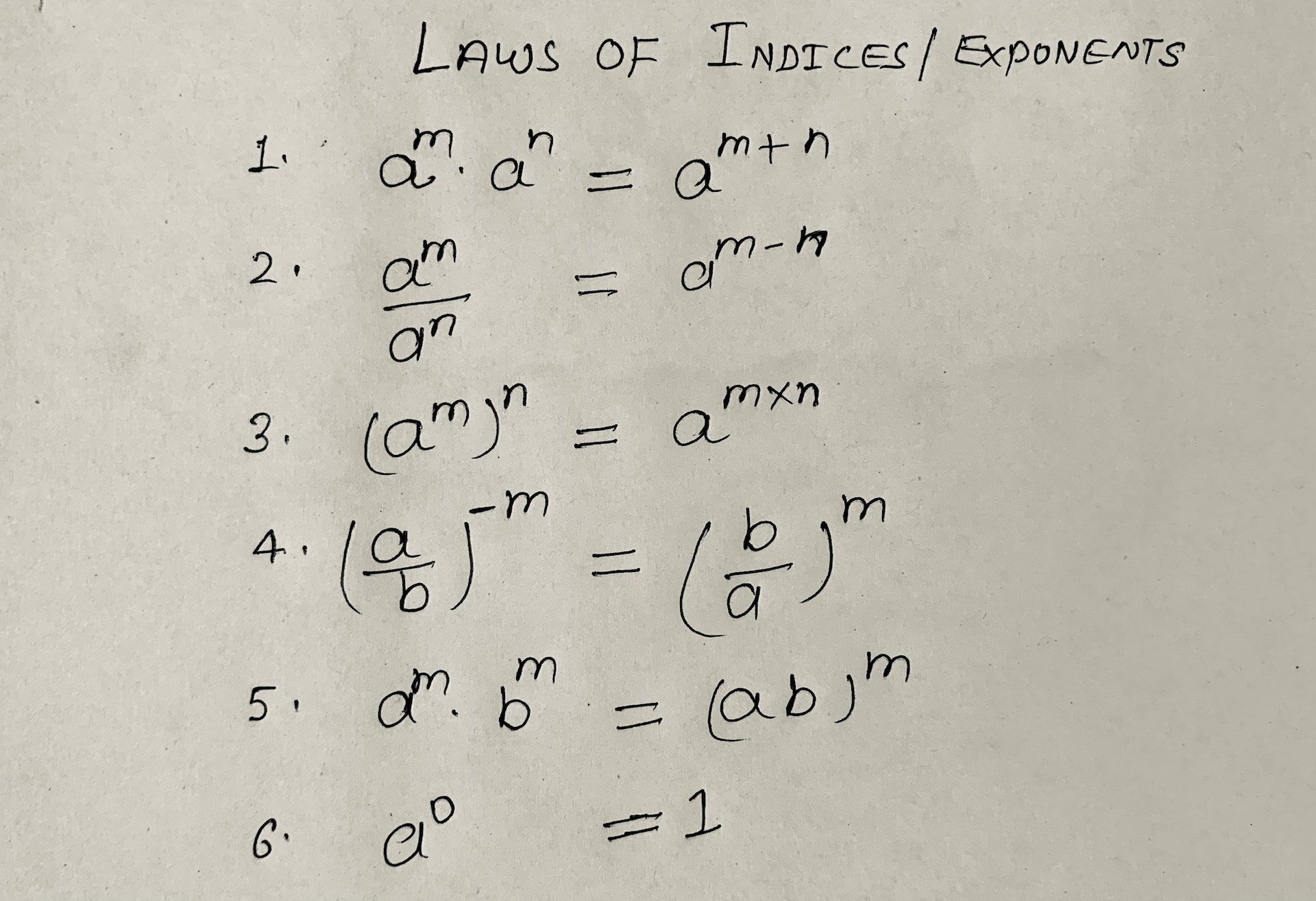# Laws of Indices or Exponents with Examples

In mathematics indices or index is the value that is raised to the power of any variable or constant like x raised to power 2 i.e. x2.

Where x is a variable and 2 is called the power or exponent of that variable.

Let’s see an example:

axm

Here

a = coefficient

x =  base

m = power or exponent or index of x

Example:

3x5 (power)

3 = coefficient,

x = base and

5 = power or exponent

When the place of variable (x) deals with numerical values like:

5m or 6

Here bases are 5 and 6 respectively and powers m and 3 are known as the index (plural form indices).

## Laws of Indices### Rule 1

When the base is the same powers will be added.

am. an = am+n
Example:

25+23 = 25+3

am÷an = am-n

Example 1:

25÷23

= 25-3

= 22

= 4

Example 2:

31/2 ÷3-1/3

= 31/2-(-1/3)

= 31/2+1/3

= 3(3+2)/3

= 35/2

(am)n = am×n

Example:

(23)2 = 23×2

= 26

### Rule 4

When the base numerator and denominator interchange with each other then the sign of the exponent will change, + becomes – and – becomes +.

(a÷b)-m = (b÷c)

or

(a÷b)m = (b÷c)-m

Example 1:

(2÷3)-5 = (3÷2)5

Note: a-m = 1÷am

Example 2:

5-2 = 1÷52

### Rule 5

If the base is different and powers are the same in that situation we can write base as a product.

am bm = (ab)m

Example:

23 53=(2×5)3

= (10)3

= 1000

### Rule 6

If the power of the base is zero, the result is always 1.

a0 = 1

√x =x1/2

Example 1:

√2 = 21/2

Example 2:

√16=161/2

= (42)1/2

= 42×1/2

### Some Practice Questions

Q1. If 8x+1 = 64, then find x…………?

Solution:

8x+1 = 64

=> 8x+1=82 [if the base of both sides is the same then we can compare the powers]

x+1 = 2

=> x=2-1

=> x=1 Ans

Q2. If 5x = 3125, then find 5x-3 …….?

Solution:

5x = 3125

5x = 55 [ if the base of both sides is the same then we can compare the powers]

x = 5

5x-3 = 55-3 [put x=5]

=> 5x-3 = 55-3

=> 5x-3 = 52

=> 5x-3 = 25

Q3. If 5x+3=(25)3x-4, then find x = …….?

Solution:

5x+3 = (25)3x-4

=> 5x+3 = (52)3x-4

=> 5x+3 = 52×(3x-4) [(am)n =a m×n]

x+3 = 2(3x-4) [if the base of both sides is the same then we can compare the powers]

=> x+3 = 6x-8

=> x-6x = -8-3

=> -5x = -11

=> x = -11/-5

=> x = 11/5

Q4. 272x-1 = (243)3, then find x ………..?

Solution:

272x-1 = (243)3

=> (3×3×3)2x-1 = (3×3×3×3×3)3

=> (33)2x-1 = (35)3

=> 36x-3 = 315

[if the base of both sides is the same then we can compare the powers]

6x-3 = 15

=> 6x = 15+3

=>6x = 18

=> x = 18/3

=> x = 6 Ans

Q5. If 6(2x+1)÷216 =36, find the value of x….?

Solution:

6(2x+1)÷216 = 36

=> 6(2x+1)÷216 = 36

=> 6(2x+1)÷63 = 62

=> 6(2x+1)-3 = 6[am÷an = am-n]

(2x+1)-3 = 2 [if the base of both sides is the same then we can compare the powers]

=> 2x+1 = 2+3

=> 2x = 5-1

=> x = 4/2

=> x = 2 Ans

Q6. Simplify the following:
(i) 1/(216)-2/3 + 1/(256)-3/4  + 1/(243)-1/5

(ii) (2/3)-2×(1/5)-2×(1/6)-2

Solution:

(i) 1/(216)-2/3 + 1/(256)-3/4  + 1/(243)-1/5

= (216)2/3 + (256)3/4 +(243)1/5        [ 1/a-m =am ]

= (63)2/3 + (44)3/4 +(35)1/5              [  (am)n=am×n  ]

= 63×2/3 +44×3/4 + 35×1/5

= 62 + 43 +3

=36 + 64+3

= 103

(ii) (2/3)-2×(1/5)-2×(1/6)-2

= (3/2)2×(5/1)2×(6/1)2

= [3/2 × 5×6 ]2

= (45)2

= 2025

When two or more exponents have addition and subtraction signs between them at that time can take common.

Example:

3x – 3x+3

=> 3x – 3x33

=>3x(1-33)

= 3x(1-27)

= 3x(-26)

Q7. If (497-496+495) = k.495 then find the value of k ….?

Solution:

(497-496+495) = k.495

=> (495+2-495+1+495) = k.495

=> (495.42-495.4+495 ) = k.495

=> 495(42-4+1) = k.495

=> 16-4+1 = k

=> 13 = k

Q8. If 2x-1+2x+1=320, then find x=…?

Solution:

2x-1+2x+1 = 320

[in this situation we will take common in L.H.S. part]

=> 2x2-1+2x21 = 320       [ by  am. an=am+n]

=> 2x[2-1+21] = 320

=> 2x[1⁄2+21] = 320

=> 2x[(1+4)÷2] = 320

=> 2x[5÷2] = 320

=> 2x = 320×2⁄5

=> 2x = 320×2⁄5

=> 2x = 64×2

=> 2x = 128

=> 2x = 27

[If the base is the same, the exponent can be compared]

=> x=7 Ans

Q9. If 2x+4-2x+2=3 ,then find x ….?

Solution:

2x+4-2x+2 = 3

=> 2x24-2x22 = 3

=> 2x24-2x22 = 3     [In this situation we will take common in L.H.S. part]

=> 2x(24 – 22) = 3

=> 2x(16-4) = 3

=> 2x(12) = 3

=> 2x(12) = 3

=> 2x = 3⁄12

=> 2x = 1⁄4

=> 2x = 1⁄22

=> 2x = 2-2      [If the base is the same, the exponent can be compared]

x = -2 Ans

Q10. If 2x-2x-1=16, then find x……?

Solution:

2x-2x-1 = 16

=> 2x-2x2-1 = 2×2×2×2

=> 2x(1-2-1) = 24

=> 2x(1-1⁄2) = 24

=> 2x(2-1)/2 = 24

=> 2x1⁄2 = 24

=> 2x = 242⁄1

=> 2x = 25              [If the base is the same, the exponent can be compared]

=> x = 5 Ans

I hope you understood about laws of indices or exponents. If still, you have any doubts, you can ask in the comment section below.